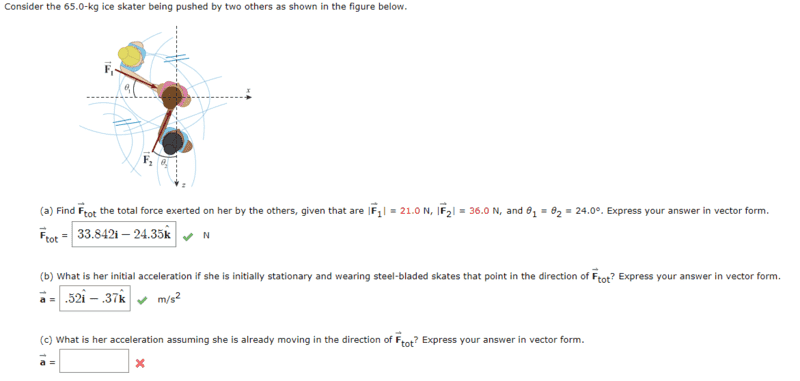# Find Her Acceleration (2 ice skaters pushing a 3rd skater)

• Austin Gibson
In summary, the student attempted to solve the homework problem, but was stumped. He submitted an equation to the teacher that the teacher accepted. The student has a rudimentary understanding of Newton's Second Law.

## Homework Statement

:
[/B]
It may be impossible to solve without the picture. Therefore, I linked the picture instead of typing the question.I'm stuck on the third question.
The third question is the following: "What is her acceleration assuming she is already moving in the direction of Ftot? Express your answer in vector form."
Notice that I already solved for her acceleration if she was stationary. Also, I'm assuming friction is disregarded because it accepted my previous answer and I disregarded friction in my calculations.

## Homework Equations

:
[/B]
F=ma

3. The Attempt at a Solution :

I'm puzzled by the question. If someone could rephrase it or nudge me towards the first step by a question, I'd be grateful. It's already shown in the picture, but I calculated her acceleration to be "a = 0.52i -0.37k" when initially stationary.

#### Attachments

Last edited by a moderator:
Does the acceleration depend on the velocity in this case? If so how?

kuruman said:
Does the acceleration depend on the velocity in this case? If so how?

Her velocity and acceleration before she's pushed are unlisted, but the net acceleration in the vertical direction is zero (otherwise, she'd be rising or falling through the ice) and, if friction and air resistance are ignored, her acceleration in horizontal before she's pushed is zero too. Therefore, she's traveling at a constant velocity and acceleration is zero. Are the answers identical or am I typing nonsense?

Last edited:
Part (c) is a variant of part (b). In part (b) you are asked to find her acceleration if she is initially at rest. You answered that correctly. In part (c) all that has changed from (b) is that she is already moving in the direction of the net force when that net force is applied. The question is, what is her acceleration now? Reason it out.

kuruman said:
Part (c) is a variant of part (b). In part (b) you are asked to find her acceleration if she is initially at rest. You answered that correctly. In part (c) all that has changed from (b) is that she is already moving in the direction of the net force when that net force is applied. The question is, what is her acceleration now? Reason it out.
After pondering about it for a few hours, I submitted my answer to (b) in (c) and it accepted it. Is my reasoning justified?

Your reasoning is OK, but you could have said better with an equation, the deceptively simple Newton's Second Law, ##\vec F_{net}=m\vec a## which says that if you know the net force, you can find the acceleration and vice versa. Velocity is not part of the picture. Think of a mass in projectile motion: it could be moving in any direction with any velocity, but its acceleration will always be g down and the net force on it (neglecting air resistance) will always be mg down.

•Austin Gibson
kuruman said:
Your reasoning is OK, but you could have said better with an equation, the deceptively simple Newton's Second Law, ##\vec F_{net}=m\vec a## which says that if you know the net force, you can find the acceleration and vice versa. Velocity is not part of the picture. Think of a mass in projectile motion: it could be moving in any direction with any velocity, but its acceleration will always be g down and the net force on it (neglecting air resistance) will always be mg down.
I'll ponder that. I was introduced to Newton's laws recently and I'm still contemplating the various implications of them. That equation is simple, but the implications are profound. It's already changing my perception of my environment.

The more proficient you become interpreting equations, the deeper your conceptual understanding.

•Austin Gibson